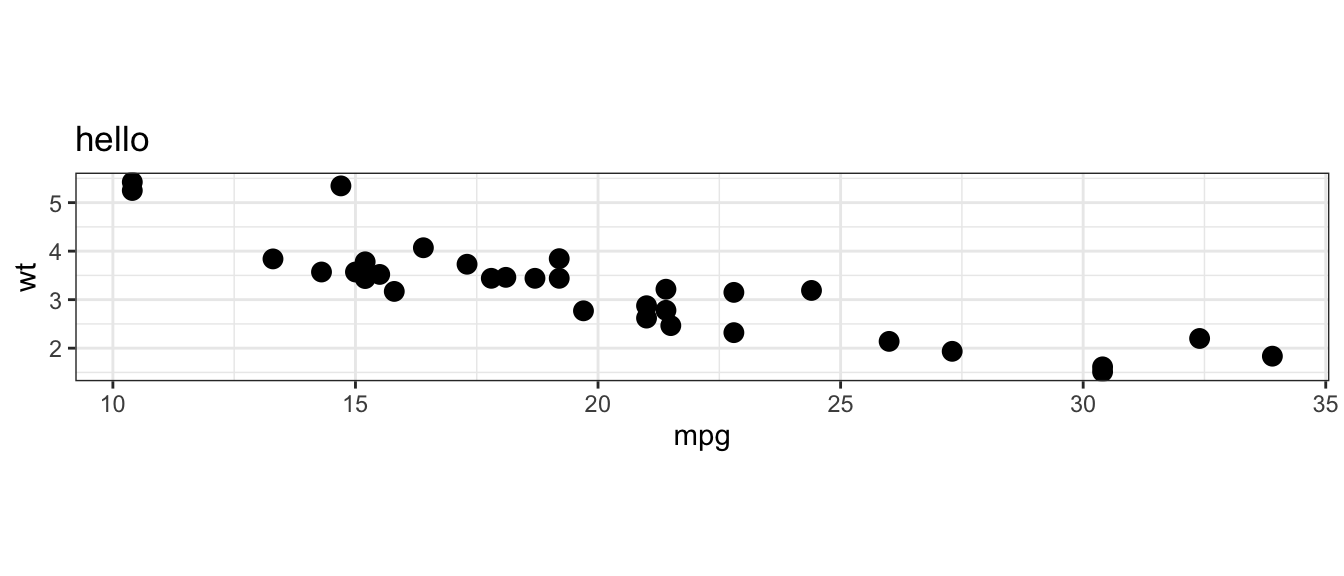# ggreverse`ggreverse` takes a ggplot object and returns the code to create that plot.

This package was written as a learning exercise to help me figure out some of the internal structure of a ggplot object.

## ToDo

• Reverse engineering of facetting and scales from a plot object.
• `aes_string()` is currently unsupported.
• Using tidyeval in `aes()` calls is currently unsupported.
• Lots of other stuff :)

## Installation

You can install from GitHub with:

``````# install.packages("remotes")
remotes::install_github("coolbutuseless/ggreverse")``````

## Example `ggreverse::convert_to_code()`

1. Create a ggplot
2. Convert the ggplot back into code using `ggreverse`
3. Convert the code back into a plot
``````library(ggreverse)

plot_df <- mtcars

# Create a ggplot2 plot object
p <- ggplot(plot_df) +
geom_point(aes(mpg, wt), size = 3) +
labs(title = "hello") +
theme_bw() +
coord_equal()````````````# Convert the plot object back into code
plot_code <- ggreverse::convert_to_code(p)
plot_code``````
``````Warning: Could not use colored = TRUE, as the package prettycode is not
installed. Please install it if you want to see colored output or see `?
``````ggplot(data = plot_df) +
geom_point(mapping = aes(x = mpg, y = wt), size = 3, position = position_identity(), stat = "identity") +
labs(title = "hello", x = "mpg", y = "wt") +
theme_bw(11) +
coord_fixed()``````
``````# Parse the plot code back into a plot - which should match the original plot
eval(parse(text = plot_code))``````## SessionInfo

Developed against:

• R 3.5.3
• ggplot2 v3.1.1